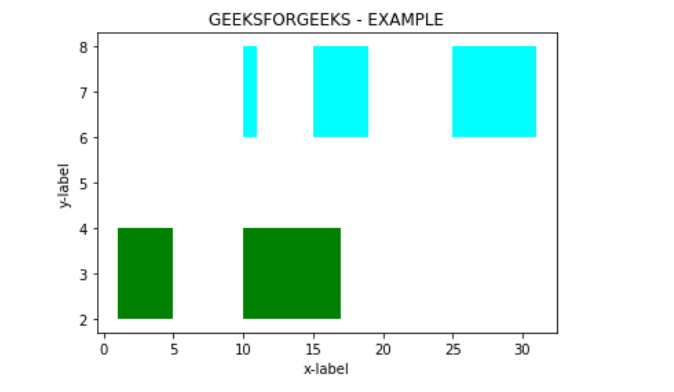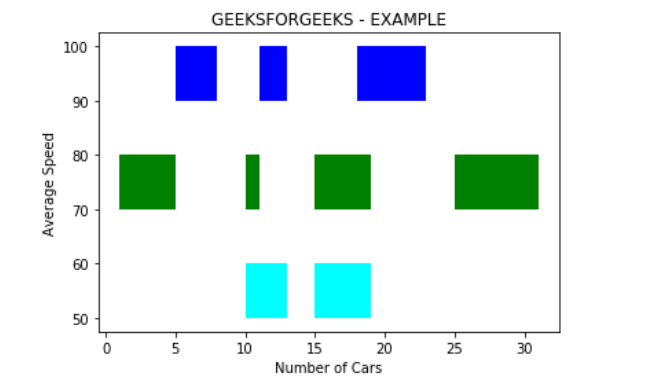# Matplotlib.pyplot.broken_barh() in Python

• Last Updated : 21 Apr, 2020

Matplotlib is one of the most popular Python packages used for data visualization. It is a cross-platform library for making 2D plots from data in arrays. Pyplot is a collection of command style functions that make matplotlib work like MATLAB.

## matplotlib.pyplot.broken_barh()

The function broken_barh() is used to Plot a horizontal sequence of rectangles.A rectangle is drawn for each component of xranges which consists of a sequence of tuples. All rectangles have the same vertical position and estimate characterized by yrange.

Attention geek! Strengthen your foundations with the Python Programming Foundation Course and learn the basics.

To begin with, your interview preparations Enhance your Data Structures concepts with the Python DS Course. And to begin with your Machine Learning Journey, join the Machine Learning - Basic Level Course

Syntax: matplotlib.pyplot.broken_barh(xranges, yrange, *, data=None, **kwargs)

Parameters:

• xranges : sequence of tuples (xmin, xwidth)
Each tuples gives the position(xmin) of the rectangle and it’s horizontal extension(xwidth) from that position.
• yranges : (ymin, ymax)
In the above attribute, ymin gives the position of the rectangle and ymax gives the vertical extension from ymin.

Returns:

• BrokenBarHCollection: A collection of horizontal bars spanning yrange with a sequence of xranges.

Examples to illustrate the matplotlib.pyplot.broken_barh() function are as follows:
Example 1:

 `# importing module``import` `matplotlib.pyplot as plt`` ` ` ` `# Adding title to the plot``plt.title(``'GEEKSFORGEEKS - EXAMPLE'``)`` ` `# adding x axis label to the plot``plt.xlabel(``'x-label'``)`` ` `# label for y axis  for the plot``plt.ylabel(``'y-label'``)`` ` `x_1 ``=` `[(``1``, ``4``), (``10``, ``7``)]``y_1 ``=` `(``2``, ``2``)`` ` `# Plotting the chart``plt.broken_barh(x_1, y_1, facecolors ``=``'green'``)`` ` `x_2 ``=` `[(``10``, ``1``), (``15``, ``4``), (``25``, ``6``)]``y_2 ``=` `(``6``, ``2``)`` ` `# Plotting the chart``plt.broken_barh(x_2, y_2, facecolors ``=``'cyan'``)`` ` `plt.show()`

Output :Example 2:

 `# importing module``import` `matplotlib.pyplot as plt`` ` ` ` `# Adding title to the plot``plt.title(``'GEEKSFORGEEKS - EXAMPLE'``)`` ` `# adding x axis label to the plot``plt.xlabel(``'Number of Cars'``)`` ` `# label for y axis  for the plot``plt.ylabel(``'Average Speed'``)`` ` `x_1 ``=` `[(``10``, ``3``), (``15``, ``4``)]``y_1 ``=` `(``50``, ``10``)`` ` `# Plotting the chart``plt.broken_barh(x_1, y_1, facecolors ``=``'cyan'``)`` ` `x_2 ``=` `[(``1``, ``4``), (``10``, ``1``), (``15``, ``4``), (``25``, ``6``)]``y_2 ``=` `(``70``, ``10``)`` ` `# Plotting the chart``plt.broken_barh(x_2, y_2, facecolors ``=``'green'``)`` ` `x_3 ``=` `[(``5``, ``3``), (``11``, ``2``), (``18``, ``5``)]``y_3 ``=` `(``90``, ``10``)`` ` `# Plotting the chart``plt.broken_barh(x_3, y_3, facecolors ``=``'blue'``)`` ` `plt.show()`

Output :My Personal Notes arrow_drop_up